# How to write product(Π) and big Pi by prod command in LaTeX?

Product notation is represented by the Greek alphabet pi(Π). Many of you think cross notation is product notation but not.

Default \prod command in latex is defined for this pi notation. You will see two uses of the \prod command in latex based on the location of the limit.

## Product symbol with both limits in LaTeX

The command for displaying a product symbol is \prod and the general syntax for typesetting products with limits in LaTeX is

\prod_{min}^{max}

which types a product with a lower limit min and upper limit max.

\documentclass{article}
\usepackage{amsmath}
\begin{document}
$\prod_{i=1}^{n}x_iy_i = \left(\prod_{i=1}^nx_i\right)\left(\prod_{i=1}^ny_i\right)\quad \text{and} \quad \left(\prod_{i=1}^{n}x_i\right)^a = \prod_{i=1}^nx_i^a$
$\prod_{i=m}^{\infty}x_i = \lim_{n \to \infty}\pi_{i=m}^{n}x_I\quad \text{and}\quad \prod_{t = -\infty}^{\infty}x_i = \left(\lim_{m \to -\infty}\prod_{i=m}^{0}x_i\right) \cdot \left(\lim_{n \to \infty}\prod_{i=1}^{n}x_i\right)$
\end{document}

Output :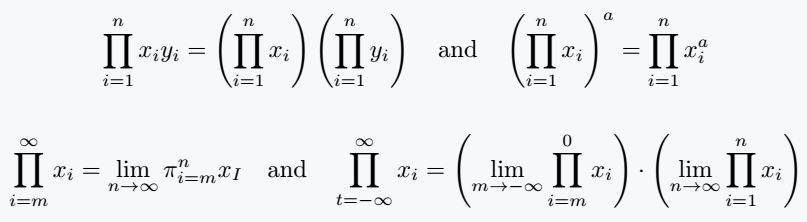Just like the integral and summation signs, the limits of the product appear differently in the inline mode and the display mode.

In the inline mode, the limits appear next to the symbol and in the display mode, the limit appear below and above.

There are two commands that are capable of controlling how these limits appear.

First is  \limits command which forcefully pushes the limits below and above of this symbol in all math modes and second is  \nolimits command which reverse the effect(forces the limits to stay next to the product symbol in all math modes).\documentclass{article}
\usepackage{amsmath}
\begin{document}
In mathematics, a multiplicative structure is denoted by the capital Pi notation($\Pi$).\\

Given that $n \in \mathbb{N}^{\ast}$, the product of n numbers $x_1,\cdots, x_n$ is denoted $\prod\limits_{k=1}^nx_k$ and written
$\prod_{k=1}^nx_k = x_1 \times x_2 \times \cdots \times x_{n-1} \times x_n$
The factorial of a number $n, n \in \mathbb{N}^{\ast}$ using the this notation as
$n! = \prod\nolimits_{k=1}^nk = 1 \times 2 \times \cdots \times n \quad \text{where}\quad 0! = 1$
\end{document}

Output :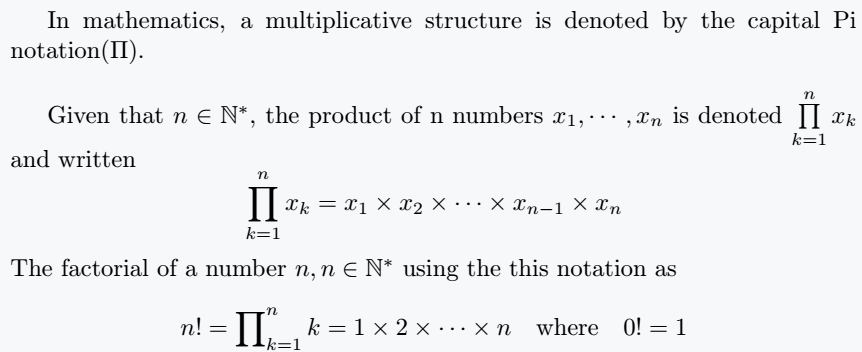## Use lower limit with \prod command

In other ways, you can only use the lower limits, not the upper and lower limits of the symbol.

Since you will use a limit, in the lower limit you will have to use conditional symbols like less than, greater than and equal, etc.

\documentclass{article}
\begin{document}
$\prod_{x=1}^{n} a_i \qquad \prod_{n\leq x \leq 1} a_i$
$\prod_{1\leq i \leq j \leq k \leq n} f_{ijk}$
$\prod_{1\leq i,k \leq j} f{\left(x_{ij}\right)}$
\end{document}

Output :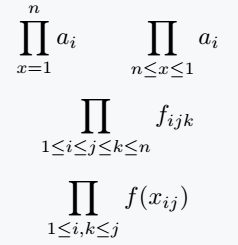In above output, spaces around the limit function can become very large for long indices and the mathematics may not be beautiful or presentable.

LaTeX has addressed this problem by providing to commands to regulate and maintain spacing making the mathematics more presentable.

Below is how to use the \smashoperator{operator with limits} and \mathclap{math} commands. Just to emphasize, this commands apply same to summations and integrals.

We apply the two commands respectively to reduce the spacing between the index and fijk as follows:

\documentclass{article}
\usepackage{mathtools}
\begin{document}
$A = \smashoperator{\prod_{1\leq i \leq j \leq k \leq n}} f_{ijk}$
$A = \prod_{\mathclap{1\leq i \leq j \leq k \leq n}} f_{ijk}$
\end{document}

Output :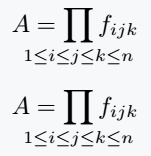The \smashoperator{operator with limits} gives you a possibility to eliminate space either just from the left(l) or right(r). Below is how to achieve that.

\documentclass{article}
\usepackage{mathtools}
\begin{document}
$A = \smashoperator[r]{\prod_{1\leq i \leq j \leq k \leq n}} B_{ijk}$
$A = \smashoperator[l]{\prod_{1\leq i \leq j \leq k \leq n}} B_{ijk}$
\end{document}

Output :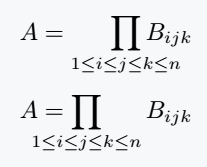You can clearly see the difference above! However, be sure to use the mathtools package for both commands.#### Md Jidan Mondal

LaTeX expert with over 10 years of experience in document preparation and typesetting. Specializes in creating professional documents, reports, and presentations using LaTeX.